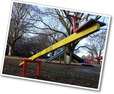## Equations and Inequalities

Type of Unit: Concept

# Prior Knowledge

Students should be able to:

• Add, subtract, multiply, and divide with whole numbers, fractions, and decimals.
• Use the symbols <, >, and =.
• Evaluate expressions for specific values of their variables.
• Identify when two expressions are equivalent.
• Simplify expressions using the distributive property and by combining like terms.
• Use ratio and rate reasoning to solve real-world problems.
• Order rational numbers.
• Represent rational numbers on a number line.

# Lesson Flow

In the exploratory lesson, students use a balance scale to find a counterfeit coin that weighs less than the genuine coins. Then continuing with a balance scale, students write mathematical equations and inequalities, identify numbers that are, or are not, solutions to an equation or an inequality, and learn how to use the addition and multiplication properties of equality to solve equations. Students then learn how to use equations to solve word problems, including word problems that can be solved by writing a proportion. Finally, students connect inequalities and their graphs to real-world situations.

Subject:
Mathematics, Algebra
Level:
Middle School
6
Tags:
• Equations
• Inequalities
• Multiplication
• Balance scale (1 for teacher demonstration) (Lesson 1)

Unit 5 Equations and Inequalities••••••••••••